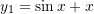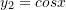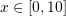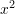### Python Libraries: Matplotlib

Matplotlib is a Python graphing library. To use Matplotlib, it must first be imported as follows:

import matplotlib.pyplot as plt
• Line Plot: plt.plot([x values],[y values])
• Scatter Plot: plt.scatter([x values],[y values])
• Title: plt.title("title")
• X-axis Label: plt.xlabel("x axis")
• Y-axis Label: plt.ylabel("y axis")
• Gridlines: plt.grid()
• Legend: plt.plot([x values],[y values],label="plot 1"); plt.legend()
• Show Plot: plt.show()

### Using Numpy

Numpy’s linspace and arange functions are usually used to generate the x values

For example, plotandon the same plot over the domain:

import numpy as np
import matplotlib.pyplot as plt

x = np.arange(0,10,0.01)
y1 = np.sin(x) + x
y2 = np.cos(x)
plt.plot(x, y1, label="y1")
plt.plot(x, y2, label="y2")
plt.title("y1 vs y2")
plt.xlabel("x")
plt.ylabel("y1 and y2")
plt.legend()
plt.grid()
plt.show()

For example, plotover the domainas a scatter graph and a line graph on separate plots

import numpy as np
import matplotlib.pyplot as plt

x = np.arange(0,10,0.5)
y = x**2

plt.plot(x, y)
plt.title("Line Graph")
plt.xlabel("x")
plt.ylabel("y")
plt.show()

plt.scatter(x, y)
plt.title("Scatter Graph")
plt.xlabel("x")
plt.ylabel("y")
plt.show()

### Using Sympy

For example, plotandon the same plot over the domainas Sympy expressions:

import numpy as np
import sympy as sp
import matplotlib.pyplot as plt

x = sp.symbols('x')
y1 = sp.sin(x) + x
y2 = sp.cos(x)
x_val = np.arange(0,10,0.01)
plt.plot(x_val, [y1.subs({x:i}) for i in x_val], label="y1")
plt.plot(x_val, [y2.subs({x:i}) for i in x_val], label="y2")
plt.title("y1 vs y2")
plt.xlabel("x")
plt.ylabel("y1 and y2")
plt.legend()
plt.grid()
plt.show()

For example, plot the Sympy expressionover the domainas a scatter graph and a line graph

import numpy as np
import sympy as sp
import matplotlib.pyplot as plt

x = sp.symbols('x')
y = x**2
x_val = np.arange(0,10,0.5)

plt.plot(x_val, [y.subs({x:i}) for i in x_val])
plt.scatter(x_val, [y.subs({x:i}) for i in x_val])
plt.title("Line and Scatter Graphs")
plt.xlabel("x")
plt.ylabel("y")
plt.show()

### Using Raw Data

For example, plot the following data points as a line graph and a scatter graph:

Data = [[0,21],[1,12],[2,5],[3,0],[4,-3],[5,-4],[6,-3],[7,0],[8,5],[9,12],[10,21]]

import matplotlib.pyplot as plt

Data = [[0,21],[1,12],[2,5],[3,0],[4,-3],[5,-4],
[6,-3],[7,0],[8,5],[9,12],[10,21]]
x_val = [point for point in Data]
y_val = [point for point in Data]

plt.plot(x_val, y_val)
plt.scatter(x_val, y_val)
plt.show()